# VI.   Rocket Nozzles:  Connection of Flow to Geometry

We have considered the overall performance of a rocket and seen that is directly dependent on the exit velocity of the propellant.  Further, we have used the steady flow energy equation to determine the exhaust velocity using the combustion chamber conditions and the nozzle exit pressure.  In this brief section, we will apply concepts from thermodynamics and fluids to relate geometrical (design) parameters for a rocket nozzle to the exhaust velocity.

We will make the following assumptions:

1. The propellant gas obeys the perfect gas law
2. The specific heat is constant
3. The flow in the nozzle is one-dimensional
4. There are no losses due to friction
5. There is no heat transfer
6. The flow velocity in the combustion chamber is negligible (zero)

## A.Quasi-one-dimensional compressible flow in a variable area duct

 p = rRT (ideal gas)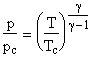(isentropic flow)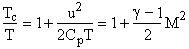(energy equation)

imply thatThen from conservation of mass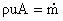(cons. of mass)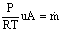The above equation relates the flow area, the mass flow, the Mach number and the stagnation conditions.  It is frequently rewritten in a non-dimensional form by dividing through by the value at M=1 (where the area at M=1 is A*):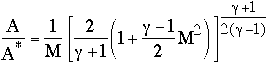which takes a form something like that shown in Figure 6.1 below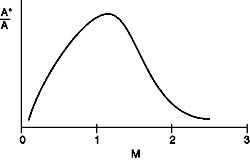Figure 6.1 General form of relationship between flow area and Mach number.

## B. Thrust in Terms of Nozzle Geometry

We can use these equations to rewrite our expression for rocket thrust in terms of  nozzle geometry (throat area = A*, and exit area Ae).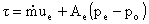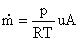evaluate at M = 1   (throat)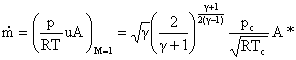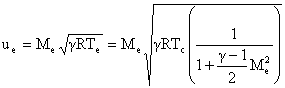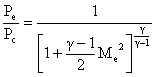We can now specify geometry (A* and Ae) to determine Me.  Then use Me with the combustion chamber conditions to determine thrust and Isp.### Interested in seeing the different types of nozzles out there? - GO!

 << Previous Unified Propulsion Next >>